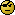## Expected power answer [Power / Sample Size]

Dear BE-proff, dear All,

❝ Let's say I want to calculate sample size based on results of a previous study.

❝ I have the following data:

❝ n=20

❝ CV=0.18 (for Cmax and AUC)❝ GMR1 = 0.97

❝ GMR2=1.19

Taking into account that CV and GMR's of such a previous (pilot) trial are not the true values but estimates with uncertainty, as ElMaestro already pointed out. One answer to such a goal is using the so-called "expected power" implemented in PowerTOST::exppower.TOST() and expsampleN.TOST().

Let's play with your numbers step by step:
1. Taking into account uncertainty of CV, but assuming a known (true) GMR =0.95
expsampleN.TOST(CV=0.18, theta0=0.95, prior.parm = list(m=20, design="2x2"), prior.type="CV") ++++++++++++ Equivalence test - TOST ++++++++++++        Sample size est. with uncertain CV ------------------------------------------------- Study design:  2x2 crossover log-transformed data (multiplicative model) alpha = 0.05, target power = 0.8 BE margins = 0.8 ... 1.25 Ratio = 0.95 CV = 0.18 with 18 df Sample size (ntotal)  n   exp. power 18   0.823287 
Not so much more than using the conventional power assuming GMR and CV known.

2. Taking into account uncertainty of CV, but assuming a true GMR =1.19
expsampleN.TOST(CV=0.18, theta0=1.19, prior.parm = list(m=20, design="2x2"), prior.type="CV") ++++++++++++ Equivalence test - TOST ++++++++++++        Sample size est. with uncertain CV ------------------------------------------------- Study design:  2x2 crossover log-transformed data (multiplicative model) alpha = 0.05, target power = 0.8 BE margins = 0.8 ... 1.25 Ratio = 1.19 CV = 0.18 with 18 df Sample size (ntotal)  n   exp. power 180   0.801466 
Again slightly higher than using the conventional power.

3. Now taking into account uncertainty of CV and GMR =1.19
expsampleN.TOST(CV=0.18, theta0=1.19, prior.parm = list(m=20, design="2x2"), prior.type="both") ++++++++++++ Equivalence test - TOST ++++++++++++   Sample size est. with uncertain CV and theta0 ------------------------------------------------- Study design:  2x2 crossover log-transformed data (multiplicative model) Design characteristics: df = n-2, design const. = 2, step = 2 alpha = 0.05, target power = 0.8 BE margins = 0.8 ... 1.25 Ratio = 1.19 with 18 df CV = 0.18 with 18 df Upper bound of expected power = 0.802418 Sample size search (ntotal)  n   exp. power Search for improved starting value based on nct approximation for conditional power: 4130   0.769089 4146   0.769157 4162   0.769219 4194   0.769354 4258   0.769619 4386   0.770131 ... 619480   0.800000 Final search: 619480   0.800000 619478   0.800000 619476   0.800000 3 iterations 619478   0.800000

That result (!) should everyone convince that using the GMR from pilot studies with small number of subjects (or likewise from usually small stage 1 of a TSD) is not a good idea, as our captainalready stated in his post above.
It results mainly from "... there is 50% chance the true GMR is worse." And power is heavily influenced by deviations in the GMR as we already know from the power analysis functions f.i. pa.ABE()

BTW: Don't ask me for the theory behind expected power. It is something Bayesian.
If you are interested you may find a short tractatus at
https://github.com/Detlew/PowerTOST/tree/master/inst/doc
written by Ben (Benjamin Lang) who is also responsible for the implementation.

Regards,

DetlewIng. Helmut Schütz